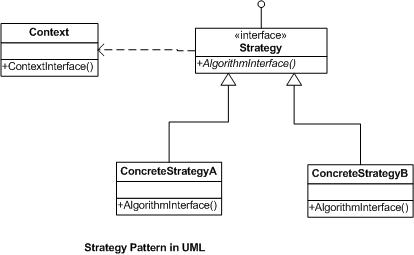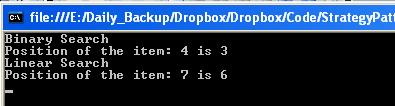# Strategy design pattern with C#

Strategy design pattern is a behavioral design pattern. It is a particular software design pattern where algorithms are selected at runtime.

According to the book of Design Pattern (Gang of Four) - “Define a family of algorithms, encapsulate each one, and make them interchangeable. Strategy lets the algorithm vary independently from clients that use it. “

The key phrases of definition are “Family of algorithms”, “encapsulate”, and “interchangeable”. Actually, Strategy Pattern encapsulates a collection of functions that do similar yet not identical jobs. Client is not bound to call fixed methods; rather it can change its strategy dynamically at run time. Client don’t call any methods directly by instantiating concrete classes. It sets its strategy via context class.

There are three main parts in strategy pattern:

• Strategy – An interface that defines how the algorithm will be called.
• Concrete Strategy – The implementation of the strategy.
• Context – It holds the concrete strategy.Implementation:

Suppose you have two lists of items. Item here integer numbers. Now, if you want to search an item from either of the lists. You can use either one of the algorithms from Linear Search or Binary search. Since binary search algorithm cannot search data without sorted list, we take here a sorted list. Now the strategy of client to use which one – Binary search or linear search. Let’s implement the problem by strategy pattern using c#.

Step 1: Create an Interface for Strategy

``````using System;
using System.Collections.Generic;
using System.Linq;
using System.Text;

namespace StrategyPattern
{
/// Strategy defines how algorithm will be called
public interface ISearchStrategy
{
int Search(int[] list, int item);
}
}

``````

Step 2: Create concrete strategy (Linear Search)

``````using System;
using System.Collections.Generic;
using System.Linq;
using System.Text;

namespace StrategyPattern
{
/// Concrete strategy(Linear Search Algorithm)
public class LinearSearch : ISearchStrategy
{
public int Search(int[] list, int item)
{
Console.WriteLine("Linear Search");
int position = 0;

for (int i = 0; i < list.Count(); i++)
{
if (list[i] == item)
{
position = i;
break;
}
}

return position;
}
}
}
``````

Step 3: Create concrete strategy (Binary Search)

``````using System;
using System.Collections.Generic;
using System.Linq;
using System.Text;

namespace StrategyPattern
{
/// Concrete strategy(Binary Search Algorithm)
public class BinarySearch : ISearchStrategy
{
public int Search(int[] list, int item)
{
Console.WriteLine("Binary Search");

int beg = 0;
int end = list.Count() - 1;
int mid = (int)((beg + end)/2);
int position = 0;

while (beg <= end && list[mid] != item)
{
if(item < list[mid])
end = mid - 1;
else
beg = mid + 1;

mid = (int)((beg + end)/2);
}

if (list[mid] == item)
position = mid;
else
position = 0;

return position;
}
}
}

``````

Step 4: Create a context class

``````using System;
using System.Collections.Generic;
using System.Linq;
using System.Text;

namespace StrategyPattern
{
/// Concrete strategy(Linear Search Algorithm)
public class LinearSearch : ISearchStrategy
{

public int Search(int[] list, int item)
{
Console.WriteLine("Linear Search");
int position = 0;

for (int i = 0; i < list.Count(); i++)
{
if (list[i] == item)
{
position = i;
break;
}
}

return position;
}
}
}

``````

Step 5: Client class to demonstrate strategy pattern

``````using System;
using System.Collections.Generic;
using System.Linq;
using System.Text;

namespace StrategyPattern
{
/// Client class
class Program
{
static void Main(string[] args)
{

int[] sortedList = { 1, 2, 3, 4, 5, 6, 7, 8 };

//Instance of context to follow different strategies
SearchList objSearchList = new SearchList();

objSearchList.SetSearchStrategy(new BinarySearch());
objSearchList.Search(sortedList, 4);

objSearchList.SetSearchStrategy(new LinearSearch());
objSearchList.Search(sortedList, 7);

}
}
}
``````

Output:Source code

Tags:

Categories:

Updated: